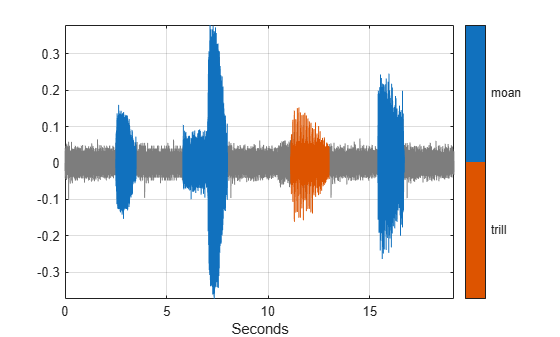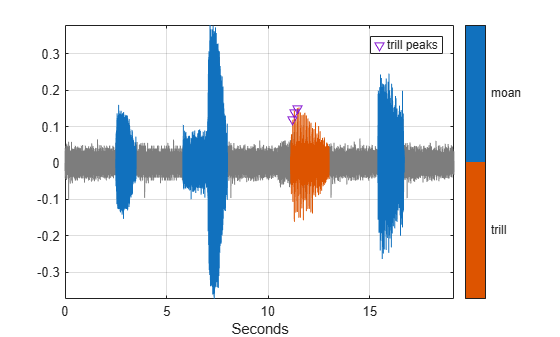# getLabeledSignal

Get labeled signals from labeled signal set

## Syntax

``[t,info] = getLabeledSignal(lss)``
``[t,info] = getLabeledSignal(lss,midx)``

## Description

example

````[t,info] = getLabeledSignal(lss)` returns a table with all the signals and labeled data in the labeled signal set `lss`.```
````[t,info] = getLabeledSignal(lss,midx)` returns a table with the signals specified in `midx`.```

## Examples

collapse all

Load a labeled signal set containing recordings of whale songs.

```load whales lss```
```lss = labeledSignalSet with properties: Source: {2x1 cell} NumMembers: 2 TimeInformation: "sampleRate" SampleRate: 4000 Labels: [2x3 table] Description: "Characterize wave song regions" Use labelDefinitionsHierarchy to see a list of labels and sublabels. Use setLabelValue to add data to the set. ```

Get a table with all the signals in `lss`.

`t = getLabeledSignal(lss)`
```t=2×4 table Signal WhaleType MoanRegions TrillRegions ________________ _________ ___________ ____________ Member{1} {79572x1 double} blue {3x2 table} {1x3 table} Member{2} {76579x1 double} blue {3x2 table} {1x3 table} ```

Identify the sublabels of the trill regions.

`d = getLabelNames(lss,'TrillRegions')`
```d = "TrillPeaks" ```

Get the labeled signal corresponding to the second member of the set. Determine the sample rate.

```idx = 2; [lbs,info] = getLabeledSignal(lss,idx)```
```lbs=1×4 table Signal WhaleType MoanRegions TrillRegions ________________ _________ ___________ ____________ Member{2} {76579x1 double} blue {3x2 table} {1x3 table} ```
```info = struct with fields: TimeInformation: "sampleRate" SampleRate: 4000 ```
`fs = info.SampleRate;`

Identify the moan and trill regions of interest. Use a `signalMask` object to plot the signal and highlight the moans and trills.

```mvals = getLabelValues(lss,idx,'MoanRegions'); tvals = getLabelValues(lss,idx,'TrillRegions'); tb = [mvals;tvals]; tb.Value = categorical( ... [repmat("moan",height(mvals),1);repmat("trill",height(tvals),1)], ... ["moan" "trill"]); sm = signalMask(tb,"SampleRate",fs); plotsigroi(sm,getSignal(lss,idx))```Identify three peaks of the trill region and plot them.

```peaks = getLabelValues(lss,idx,{'TrillRegions','TrillPeaks'}); hold on pk = plot(peaks.Location,cell2mat(peaks.Value),'v'); hold off legend(pk,'trill peaks')```## Input Arguments

collapse all

Labeled signal set, specified as a `labeledSignalSet` object.

Example: ```labeledSignalSet({randn(100,1) randn(10,1)},signalLabelDefinition('female'))``` specifies a two-member set of random signals containing the attribute `'female'`.

Member row number, specified as a positive integer. `midx` specifies the member row number as it appears in the Labels table of a labeled signal set.

## Output Arguments

collapse all

Labeled signal, specified as a table.

Time information, returned as a structure.

## Version History

Introduced in R2018b